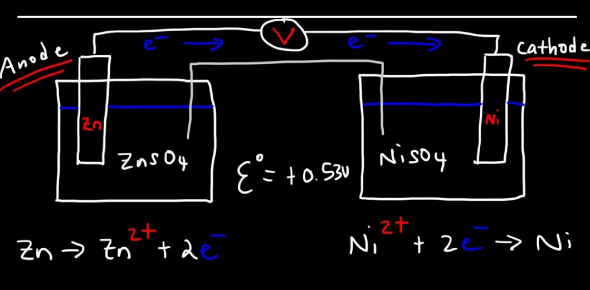# Electrochemistry MCQ Quiz Exam!

20 Questions | Attempts: 207Settings.

• 1.
What is the charge of the cations of group 2 elements?
• A.

+1

• B.

+2

• C.

+3

• D.

+4

• 2.
What is the charge of aluminum cation?
• A.

+1

• B.

+2

• C.

+3

• D.

+4

• 3.
What is the number of mole aluminum metal produced in electrolysis if 96485 C/s  of electricity is passed in 1 second?
• A.

1/3 mole

• B.

1/2 mole

• C.

1 mole

• D.

2.5 moles

• 4.
What process requires electrical energy to allow a nonspontaneous chemical redox reaction to occur?
• A.

Oxidation

• B.

Reduction

• C.

Electrolysis

• D.

Combustion

• 5.
Given the half-reaction, Fe3+(aq)   +   e-      →  Fe2+(aq)   , identify the type of chemical reaction
• A.

Reduction

• B.

Oxidation

• C.

Cathodic

• D.

Both reduction and oxidation

• E.

None of the above

• 6.
Given the galvanic cell reaction,  Cu (s) + 2Ag+ (aq) → Cu2+(aq) + 2 Ag(s)  , which is the reduced product?
• A.

Cu (s)

• B.

2Ag+ (aq)

• C.

Cu2+(aq)

• D.

2 Ag(s)

• 7.
Where does oxidation half-reaction occur in a galvanic cell?
• A.

Anode

• B.

Cathode

• C.

Salt bridge

• D.

Either cathode or anode

• 8.
What is the unit of the electric current defined as 1coulomb per second?
• A.

1 ampere

• B.

1 volt

• C.

1 coulomb

• D.

1 ohm

• 9.
Which of these metals has the highest oxidation potential in the electrochemical series?
• A.

Magnesium

• B.

Aluminum

• C.

Lithium

• D.

Copper

• 10.
If a galvanic cell is set up with Nickel as an anode, which of these can be the metal cathode?
• A.

Magnesium

• B.

Zinc

• C.

Copper

• D.

Aluminum

• 11.
What is the product of electrolysis of  molten sodium chloride at the cathode?
• A.

Chlorine gas

• B.

Sodium metal

• C.

Water vapor

• D.

Hydrogen as

• 12.
What is the charge that passes through a point when one ampere of electric current flows for one second?
• A.

1 coulomb

• B.

• C.

1 volt

• D.

1 ohm

• 13.
In the electroplating process at which metal electrode surface can aluminum deposit coming from a molten aluminum oxide?
• A.

Anode

• B.

Cathode

• C.

Either anode or cathode

• D.

Neither anode nor cathode

• 14.
What is the type of electrochemical cell that produces electricity from a spontaneous chemical redox reaction?
• A.

Electrolytic cell

• B.

Downs cell

• C.

Electroplating cell

• D.

Galvanic cell

• 15.
What is the unit measure for the difference in chemical potentials between two electrodes of an electrochemical cell?
• A.

Volt

• B.

Ohm

• C.

Ampere

• D.

Coulomb

• 16.
What is the amount of copper metal produced when one faraday of electricity is passed through a copper sulfate solution in an electrolytic cell?
• A.

2 moles

• B.

1 mole

• C.

1/2 mole

• D.

1/4 mole

• 17.
Given the electrochemical notation of a galvanic cell, Cd(s) │ Cd2+(aq)        ││  Pb2+(aq)    │ Pb(s) which is the oxidized product at one of the electrodes?
• A.

Cd(s)

• B.

Cd2+(aq)

• C.

Pb2+(aq)

• D.

Pb(s)

• 18.
Which is the anode , given the electrochemical notation of a galvanic cell,  Ni(s) │ Ni2+(aq)    ││  Ag+(aq)    │ Ag (s
• A.

Ni(s)

• B.

Ni2+(aq)

• C.

Ag+(aq)

• D.

Ag (s

• 19.
Which is is the anode of the galvanic cell, given the electrochemical notation. Fe (s) │Fe2+(aq)   ││ Cu2+(aq)  │Cu (s)
• A.

Fe (s)

• B.

Fe2+(aq)

• C.

Cu2+(aq)

• D.

Cu (s)

• 20.
Which of the following statements is correct?
• A.

Reduction occurs at the anode in both electrolytic and galvanic cell.

• B.

Oxidation occurs at the cathode in both electrolytic and galvanic cell.

• C.

Reduction occurs at the cathode in both electrolytic and galvanic cell

• D.

Oxidation occurs at the cathode of galvanic cell only.

## Related TopicsBack to top
×

Wait!
Here's an interesting quiz for you.